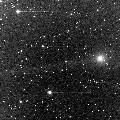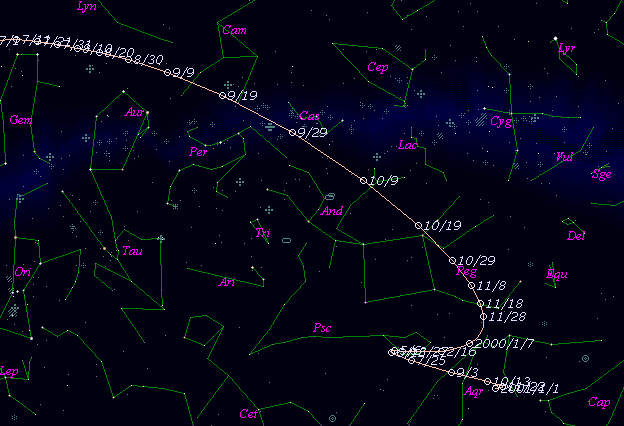# \$B%j!

C/1999 H1 ( Lee )###\$B%W%m%U%#!<%k(B

 \$BH/8+F|(B 1999\$BG/(B 4\$B7n(B16\$BF|(B \$BH/8+8wEY(B 9\$BEy(B \$BH/8+ Steven Lee (Mudgee, N.S.W.)

###\$B###\$B50F;MWAG(B

```   The following improved orbital elements by Kenji Muraoka, are from
888 observations 1999 Apr. 16 to Dec. 10, including 8 Planets, Moon
and 3 minor planets perturbations and non-gravitational effect of
style II.  The mean residual is +/- 0.78 arc seconds.

Epoch  =  1999 July  1.0  TT       JDT = 2451360.5
T  =  1999 July 11.17316       +/- 0.00019 (m.e.) TT
Peri. =   40.70436                +/- 0.00060
Node  =  162.65140                +/- 0.00018   (2000.0)
Incl. =  149.35276                +/- 0.00009
q  =    0.7080729              +/- 0.0000070 AU
e  =    0.9996221              +/- 0.0000050
1/a  =   +0.0005337              +/- 0.0000071 1/AU
A1  =   +2.65                   +/- 0.28
A2  =   -0.1028                 +/- 0.0527
( P  =  81103 years )
```

###\$B@1?^(B###\$B8wEYJQ2=(B

```        m1 = 6.3 + 5 log\$B&\$(B + 14.0 log r  [   ,-35]  (              \$B!A(B1999\$BG/(B 6\$B7n(B 6\$BF|(B)
m1 = 6.3 + 5 log\$B&\$(B +  5.0 log r  [-35, 40]  (1999\$BG/(B 6\$B7n(B 6\$BF|!A(B1999\$BG/(B 8\$B7n(B20\$BF|(B)
m1 = 6.0 + 5 log\$B&\$(B + 14.0 log r  [ 40,120]  (1999\$BG/(B 8\$B7n(B20\$BF|!A(B1999\$BG/(B11\$B7n(B 8\$BF|(B)
m1 = 8.9 + 5 log\$B&\$(B + 10.0 log r  [120,   ]  (1999\$BG/(B11\$B7n(B 8\$BF|!A(B              )
```##### \$B50F;MWAG\$OB<2,7r<#;a\$N7W;;\$K\$h\$k\$b\$N\$G\$9!#(B \$B@1?^\$O(B StellaNavigator Ver.2.0 for Windows (\$B%"%9%H%m%"!<%D(B \$BJTCx(B / \$B%"%9%-!<=PHG6I4)(B) \$B\$G:n@.\$7\$?\$b\$N\$G\$9!#(B \$B8wEY%0%i%U\$O(BComet for Windows\$B\$G:n@.\$7\$?\$b\$N\$G\$9!#(B